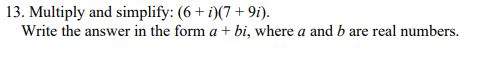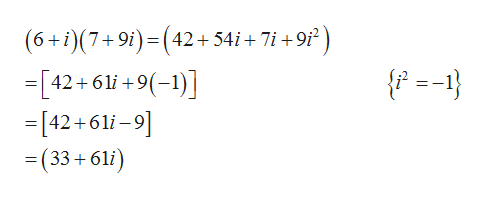# 13. Multiply and simplify: (6+ i)(7 + 9i).Write the answer in the form a + bi, where a and b are real numbers.

Question
2 views

practice question 13help_outlineImage Transcriptionclose13. Multiply and simplify: (6+ i)(7 + 9i). Write the answer in the form a + bi, where a and b are real numbers. fullscreen
check_circle

Step 1

Given expression is

Step 2

On multiply...help_outlineImage Transcriptionclose(6+i)(7+9i)=(42+54i+ 7i +9i² ) + 9i {f =-1} =[42+61i +9(-1)] =[42+61i –9] (33+61i) = fullscreen

### Want to see the full answer?

See Solution

#### Want to see this answer and more?

Solutions are written by subject experts who are available 24/7. Questions are typically answered within 1 hour.*

See Solution
*Response times may vary by subject and question.
Tagged in

### Algebra